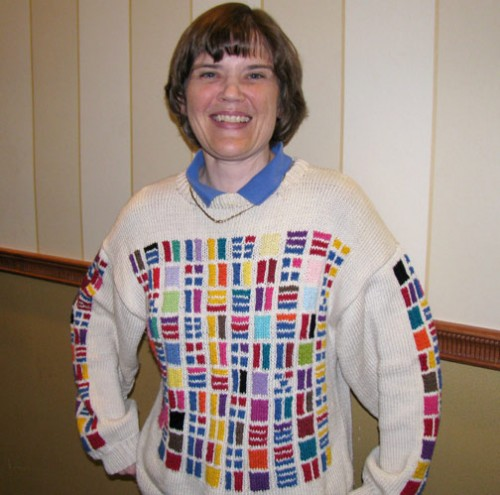Sondra Eklund, a knitter, mathematician, and YA librarian, designed and crafted this sweater. It shows, in colors, the prime factorization of every number between 2 and 100…

Okay, here’s how it works. You have to start in the bottom lefthand corner, because the mathematician in me couldn’t bear to start anywhere except where the origin would be on Cartesian coordinates. Naturally, the numbers go from left to right and from low to high.

Okay, look at the bottom row. It looks like there is a blank space on the left. That represents 1, because 1 is the background color, because 1 is a factor of every number. Next is a blue square, which represents 2. Next is a red square, for 3. Then comes 4. 4 = 2 x 2. So 4 is represented by two blue rectangles. Then comes 5. 5 is prime, so 5 gets a new color, yellow.

Next is 6. 6 = 2 x 3. So 6 is represented by a blue rectangle and a red rectangle. […] Get the idea? This sweater presents a chart giving the color-coded prime factorization of every number from 2 to 100.

Makes sense to me.